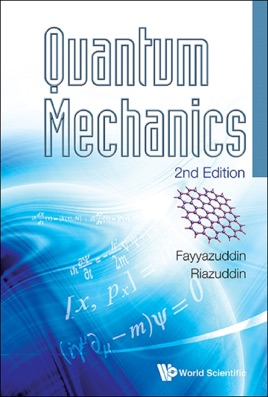• \$45.99

## Publisher Description

This book provides a comprehensive account of basic concepts of quantum mechanics in a coherent manner. The book is self-contained and not only covers basic concepts in quantum mechanics but also provides a basis for applications in atomic and laser physics, nuclear and particle physics, and condensed matter physics. It also covers relativistic quantum mechanics, in particular the Dirac equation and its applications.
Contents:Breakdown of Classical ConceptsQuantum Mechanical ConceptsBasic Postulates of Quantum MechanicsSolution of Problems in Quantum MechanicsSimple Harmonic OscillatorAngular MomentumMotion in Centrally Symmetric FieldCollision TheoryOperatorsHeisenberg Equation of Motion, Invariance Principle and Path IntegralAngular Momentum and SpinTime Independent Perturbation TheoryTime Dependent Perturbation TheoryStatistics and the Exclusion PrincipleTwo State SystemsQuantum ComputationPerturbation Induced by Electromagnetic FieldFormal Theory of ScatteringS-Matrix and Invariance PrinciplesRelativistic Quantum Mechanics: Dirac EquationDirac Equation in (1+2) Dimensions: Application to Graphene